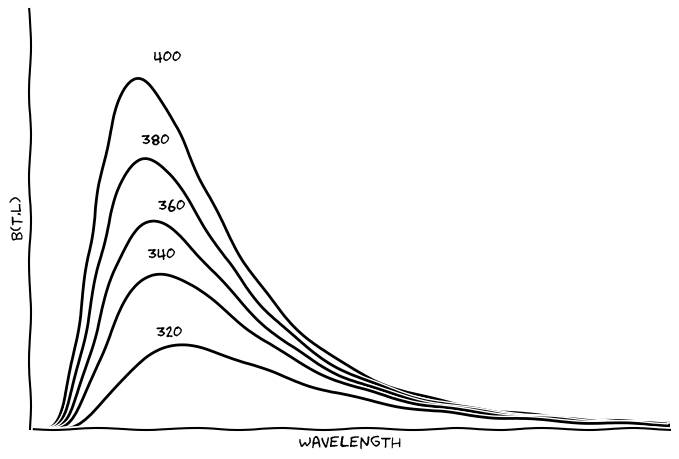## Wien's law step by step

### Mi 11 Mai 2016

The Wein's displacement law is obtained from Planck's law. Wein's law states that the product between wavelength, for a maximum emissivity, and body's temperature $$(T)$$, is a constant. In other words, if we know the body's temperature, we'll be able to determine the wavelength were the maximum emissivity occur.

The Planck's law is given by:

\begin{equation} \label{eq1} B(T,\lambda) = \frac{2hc^{2}}{\lambda^{5}(exp(\frac{hc}{kT})-1)} \end{equation}

The figure below shows a family of emissivity curves for five values of $$T$$.Hereafter, we'll show how the Wein's law cam be found.

For simplicity, let's define $$\alpha\equiv 2hc^{2}$$ and $$\gamma\equiv \frac{hc}{kT}$$

Then, the equation \ref{eq1} becomes:

\begin{equation} \label{eq2} B(\gamma, \lambda) = \alpha\lambda^{-5}(exp(\frac{\gamma}{\lambda})-1)^{-1} \end{equation}

But, what we want to find? We are interested to find in which wavelength occur the maximum emissivity. For this, we need to make $$\frac{\partial B}{\partial \lambda}=0$$.

\begin{equation} \begin{split} \frac{\partial B}{\partial \lambda}=\alpha\lambda^{-6}(exp(\frac{\gamma}{\lambda})-1) [-5+ \\ \gamma\lambda^{-1}(exp(\frac{\gamma}{\lambda})-1)^{-1}exp(\frac{\gamma}{\lambda})] =0 \end{split} \label{eq3} \end{equation}

The solution of equation \ref{eq3} is found doing the factor inside of brackets equal to zero.

\begin{equation} -5+ \gamma\lambda^{-1}(exp(\frac{\gamma}{\lambda})-1)^{-1}exp(\frac{\gamma}{\lambda}) =0 \label{eq4} \end{equation}

After reordering all the factors in \ref{eq4}, we will get the equation bellow.

\begin{equation} \lambda[\exp(\frac{\gamma}{\lambda})(\frac{\gamma}{\lambda}-5)+5]=0 \label{eq5} \end{equation}

Once that $$\lambda$$ is different of zero, the factor inside of brackets has to be zero.

\begin{equation} exp(\frac{\gamma}{\lambda})(\frac{\gamma}{\lambda}-5)+5=0 \label{eq6} \end{equation}

The equation \ref{eq6} is a transcendental equation, that means, it is not possible to solve by traditional way. For simplicity, we will define $$g\equiv \frac{\gamma}{\lambda}$$. Following, the last equation becomes

\begin{equation} exp(g)(g-5)+5=0 \label{eq7} \end{equation}

So, how to solve it? One way cam be expressing exponential function by an approximation using Taylor's series. Another way is to use graphical method (For the moment, we'll demonstrate just the first way).

\begin{equation} exp(g)=1+g+\frac{g^2}{2} \label{eq8} \end{equation}

After replacing $$\ref{eq8}$$ in $$\ref{eq7}$$; we get:

\begin{equation} (1+g+\frac{g^2}{2})(g-5)+5=0 \label{eq9} \end{equation}

After reorganize, we find that:

\begin{equation} g(-4-\frac{3g}{2}+\frac{g^2}{2})=0 \label{eq10} \end{equation}

According definition $$g$$ is different of zero; so, the expression inside of brackets has to be equal to zero. This equation is a second degree equation; then, we may conclude that $$g$$ is a constant.

Therefore,

\begin{equation} g=\frac{\gamma}{\lambda}=\frac{hc}{kT\lambda}=constant \Rightarrow {\lambda}{T}=constant \end{equation}

Quod erat demonstrandum.